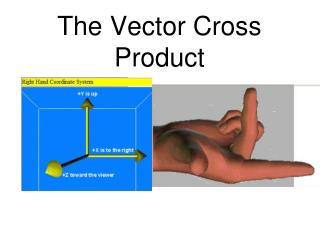DownloadDownload PresentationThe Vector Cross Product

# The Vector Cross Product

Download Presentation## The Vector Cross Product

- - - - - - - - - - - - - - - - - - - - - - - - - - - E N D - - - - - - - - - - - - - - - - - - - - - - - - - - -
##### Presentation Transcript

1. The Vector Cross Product

2. Definition The cross product of two 3-d vectors becomes a 3-d vector itself. The cross product is: Example Method 1 An easier method is to use the formula listed in the matrices part of the formula sheet. Method 2

3. Applications of vxw - the perpendicular vector The resulting vector of the cross product of vxw will always be perpendicular to v and w, as shown in the diagram below. Find the vector that is perpendicular to: 1. and 2. 3i + 2j - k and 3j - 5k. -7i + 15j + 9k

4. Applications of vxw - the area of a triangle or parallelogram Area of a triangle is given by . If a and b are vectors the area of a triangle becomes: Proof: Expand and simplify

5. So that proof means: The area of a triangle where a and b are vectors is given by:

6. Find the area of the triangle with vertices A(1,1,2) B(-1,3,2) and C(4,1,5). Find two sides of the triangle, the vectors AB and AC. The area is given as follows: and Now find the cross product of the two vectors:

7. TRY THESE YOURSELF Find the area of the triangle with vertices A(2,7,3), B(-8,3,1) and C(1,6,-2). Find two perpendicular unit vectors to the plane containing the points (4,1,3), (1,1,2) and (7,2,-4).